JSL Syntax Reference > JSL Functions > Probability Functions
Publication date: 05/24/2021

# Probability Functions

Beta Density(x, alpha, beta, <theta=0>, <sigma=1>)

Description

Returns the probability density function (pdf) evaluated at x of the beta distribution. The pdf is parameterized as follows: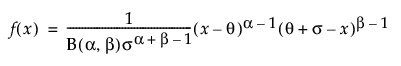where B(·) is the Beta function.

Arguments

x

A quantile at which the pdf is evaluated. x must be between theta and theta + sigma.

alpha, beta

Shape parameters α and β, which must both be greater than 0.

theta

Optional threshold parameter θ. The default is 0.

sigma

Optional scale parameter σ, which must be greater than 0. The default is 1.

Notes

The beta distribution is useful for modeling the probabilistic behavior of random variables that are constrained to fall in the interval [0, 1], such as proportions.

Beta Distribution(x, alpha, beta, <theta=0>, <sigma=1>)

Description

Returns the cumulative distribution function (cdf) evaluated at x of the beta distribution. The cdf uses the same parameterization as the Beta Density() function.

Arguments

x

A quantile at which the cdf is evaluated. x must be between theta and theta + sigma.

alpha, beta

Shape parameters α and β, which must both be greater than 0.

theta

Optional threshold parameter θ. The default is 0.

sigma

Optional scale parameter σ, which must be greater than 0. The default is 1.

Beta Quantile(p, alpha, beta, <theta=0>, <sigma=1>)

Description

Returns the pth quantile from a beta distribution with shape arguments alpha and beta. The quantile function does not have a closed form equation.

Arguments

p

The probability of the quantile desired. p must be between 0 and 1.

alpha, beta

Shape parameters α and β, which must both be greater than 0.

theta

Optional threshold parameter θ. The default is 0.

sigma

Optional scale parameter σ, which must be greater than 0. The default is 1.

Cauchy Density(q, <center=0>, <scale=1>)

Description

Returns the probability density function (pdf) evaluated at q of a Cauchy distribution. The pdf is parameterized as follows: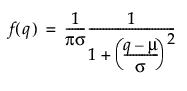Arguments

q

A quantile at which the pdf is evaluated.

center

Optional location parameter μ. The default is 0.

scale

Optional scale parameter, σ, which must be greater than 0. The default is 1.

Cauchy Distribution(q, <center=0>, <scale=1>)

Description

Returns the cumulative distribution function (cdf) probability that a Cauchy distributed random variable is less than q. The cdf is parameterized as follows: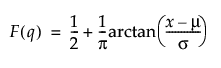Arguments

q

A quantile at which the cdf is evaluated.

center

Optional location parameter μ. The default is 0.

scale

Optional scale parameter, σ, which must be greater than 0. The default is 1.

Cauchy Quantile(p, <center=0>, <scale=1>)

Description

Returns the pth quantile from a Cauchy distribution. The pth quantile is the value for which the probability is p that a random value would be less than or equal to p. The quantile function is parameterized as follows: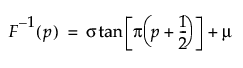Arguments

p

The probability of the quantile desired. p must be between 0 and 1.

center

Optional location parameter μ. The default is 0.

scale

Optional scale parameter σ, which must be greater than 0. The default is 1.

ChiSquare Density(q, df, <nc=0>)

Description

Returns the probability density function (pdf) evaluated at q of the chi-square distribution. The pdf is parameterized as follows: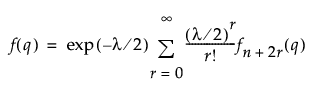where fn+2r(q) is the density of a central chi-square distribution with n+2r degrees of freedom.

Arguments

q

A quantile at which the pdf is evaluated. q must be greater than or equal to 0.

df

The degrees of freedom n, which must be greater than 0.

nc

Optional noncentrality parameter λ, which must be nonnegative. The default is 0.

ChiSquare Distribution(q, df, <nc=0>)

Description

Returns cumulative distribution function at quantile x for chi-square with df degrees of freedom centered at nc. The cdf is parameterized as follows: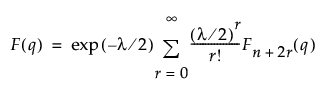,

where Fn+2r(q) is the cumulative distribution of a central chi-square distribution with n+2r degrees of freedom.

Arguments

q

A quantile at which the cdf is evaluated. q must be greater than or equal to 0.

df

The degrees of freedom n, must be greater than 0.

nc

The optional noncentrality parameter λ, must be nonnegative. The default is 0.

ChiSquare Log CDistribution(x, df, <nc=0>)

Description

Returns the log of (1 - value), where value is the cumulative distribution function evaluated at x of the chi-square distribution with df degrees of freedom and noncentrality parameter nc.

ChiSquare Log Density(x, df, <nc=0>)

Description

Returns the log of the value of the probability density function evaluated at x of the chi-square distribution with df degrees of freedom and noncentrality parameter nc.

ChiSquare Log Distribution(x, df, <nc=0>)

Description

Returns the log of the value of the cumulative distribution function evaluated at quantile x of the chi-square distribution with df degrees of freedom and noncentrality parameter nc.

ChiSquare Noncentrality(x, df, prob)

Description

Returns the chi-square distribution noncentrality parameter nc that satisfies the following:

prob = ChiSquare Distribution(x, df, nc)

Arguments

x

A quantile at which the cdf is evaluated.

df

The degrees of freedom n, which must be greater than 0.

prob

The probability of the quantile desired; prob must be between 0 and 1.

ChiSquare Quantile(p, df, <nc=0>)

Description

Returns the pth quantile from a chi-square distribution with df degrees of freedom, centered at nc. The quantile function does not have a closed form equation.

Arguments

p

The probability of the quantile desired. p must be between 0 and 1.

df

The degrees of freedom n, which must be greater than 0.

nc

Optional noncentrality parameter λ, which must be nonnegative. The default is 0.

Dunnett P Value(q, nTrt, dfe, <lambdaVec=.>)

Description

Returns the p-value from Dunnett’s multiple comparisons test.

Arguments

q

A number that is the test statistic.

nTrt

The number of treatments being compared to the control treatment.

dfe

The error degrees of freedom.

lambdaVec

A vector of parameters. If lambdaVec is missing (.), each of the parameters is set to 1/Sqrt(2).

Dunnett Quantile(1-alpha, nTrt, dfe, <lambdaVec=.>)

Description

Returns the quantile used in Dunnett’s multiple comparisons test.

Arguments

1-alpha

A number that is the confidence level.

nTrt

The number of treatments being compared to the control treatment.

dfe

The error degrees of freedom.

lambdaVec

A vector of parameters. If lambdaVec is missing (.), each of the parameters is set to 1/Sqrt(2).

Exp Density(x, <theta=1>)

Description

Returns the probability density function (pdf) evaluated at x of the exponential distribution. The pdf is parameterized as follows: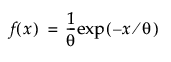Arguments

x

A quantile at which the pdf is evaluated. x must be greater than or equal to 0.

theta

Optional scale parameter θ, which must be greater than 0. The default is 1.

Exp Distribution(x, <theta=1>)

Description

Returns the cumulative distribution function (cdf) evaluated at x of the exponential distribution. The cdf is parameterized as follows: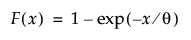Arguments

x

A quantile at which the cdf is evaluated. x must be greater than or equal to 0.

theta

Optional scale parameter θ, which must be greater than 0. The default is 1.

Exp Quantile(p, <theta=1>)

Description

Returns the pth quantile from an exponential distribution with scale parameter theta. The quantile function is parameterized as follows: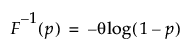Arguments

p

The probability of the quantile desired. p must be between 0 and 1.

theta

Optional scale parameter θ, which must be greater than 0. The default is 1.

F Density(x, dfnum, dfden, <nc=0>)

Description

Returns the probability density function (pdf) evaluated at x for the F distribution with numerator and denominator degrees of freedom dfnum and dfden, with optional noncentrality parameter nc.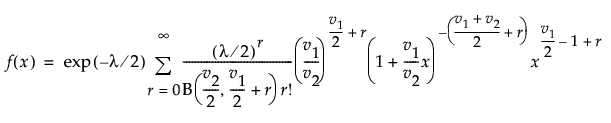where B(·) is the Beta function.

Arguments

x

A quantile at which the pdf is evaluated. x must be greater than 0.

dfnum

The degrees of freedom, v1, of the chi-square distribution in the numerator of the F-distribution. dfnum must be greater than 0.

dfden

The degrees of freedom, v2, of the chi-square distribution in the denominator of the F-distribution. dfden must be greater than 0.

nc

Optional noncentrality parameter λ, which must be nonnegative. The default is 0.

F Distribution(x, dfnum, dfden, <nc=0>)

Description

Returns the cumulative distribution function (cdf) evaluated at x for the F distribution with numerator and denominator degrees of freedom dfnum and dfden and noncentrality parameter nc.

F Log CDistribution(x, dfnum, dfden, <nc=0>)

Description

Returns the log of (1 - value), where value is the cumulative distribution function evaluated at x of the F distribution with numerator and denominator degrees of freedom dfnum and dfden, with optional noncentrality parameter nc.

F Log Density(x, dfnum, dfden, <nc=0>)

Description

Returns the log of the value of the probability density function (pdf) evaluated at x for the F distribution with numerator and denominator degrees of freedom dfnum and dfden, with optional noncentrality parameter nc.

F Log Distribution(x, dfnum, dfden, <nc=0>)

Description

Returns the log of the value of the cumulative distribution function (cdf) evaluated at x for the F distribution with numerator and denominator degrees of freedom dfnum and dfden and noncentrality parameter nc.

F Noncentrality(x, dfnum, dfden, prob)

Description

Returns the F distribution noncentrality parameter nc that satisfies the following:

prob = F Distribution(x, dfnum, dfden, nc)

F Distribution(x, dfnum, dfden, <nc=0>)

F Power(alpha, dfh, dfm, d, n)

Description

Returns the power from a given situation involving an F test or a t test.

Arguments

alpha

The significance level of the test. alpha must be between 0 and 1.

dfh

The hypothesis degrees of freedom. dfh must be greater than 0.

dfm

The degrees of freedom in the whole model. dfm must be greater than 0.

d

The squared effect size, defined as Δ2/σ2. In this equation, σ2 is the error variance and Δ2 is defined as follows: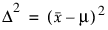for a one-sample t test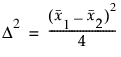for a two-sample t test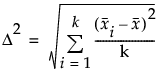for a k-sample F test

n

The total number of observations. n must be greater than dfm.

F Quantile(x, dfnum, dfden, <nc=0>)

Description

Returns the pth quantile from the F distribution with numerator and denominator degrees of freedom dfnum and dfden and noncentrality parameter nc.

F Sample Size(alpha, dfh, dfm, d, power)

Description

Returns the sample size from a given situation involving an F test or a t test.

Arguments

alpha

The significance level of the test. alpha must be between 0 and 1.

dfh

The hypothesis degrees of freedom. dfh must be greater than 0.

dfm

The degrees of freedom in the whole model. dfm must be greater than 0.

d

The squared effect size, defined as Δ2/σ2. In this equation, σ2 is the error variance and Δ2 is defined as follows:for a one-sample t testfor a two-sample t testfor a k-sample F test

power

The desired power for the test.

Frechet Density(x, mu, sigma)

Description

Returns the probability density function (pdf) evaluated at x of the Fréchet distribution. The pdf is parameterized as follows: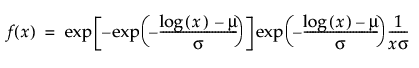Arguments

x

A quantile at which the pdf is evaluated. x must be greater than 0.

mu

The location parameter μ.

sigma

The scale parameter σ, which must be greater than 0.

Frechet Distribution(x, mu, sigma)

Description

Returns the cumulative distribution function (cdf) evaluated at x of the Fréchet distribution. The cdf is parameterized as follows: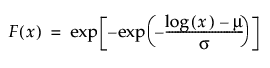Arguments

x

A quantile at which the cdf is evaluated. x must be greater than 0.

mu

The location parameter μ.

sigma

The scale parameter σ, which must be greater than 0.

Frechet Quantile(p, mu, sigma)

Description

Returns the pth quantile from a Fréchet distribution with location mu and scale sigma. The quantile function is parameterized as follows: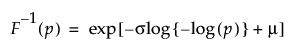Arguments

p

The probability of the quantile desired. p must be between 0 and 1.

mu

The location parameter μ.

sigma

The scale parameter σ, which must be greater than 0.

Gamma Density(x, <alpha=1>, <scale=1>, <threshold=0>)

Description

Returns the probability density function (pdf) evaluated at x of the Gamma distribution. The pdf is parameterized as follows: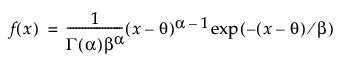Arguments

x

A quantile at which the pdf is evaluated. x must be greater than θ.

alpha

Optional shape parameter α, which must be greater than 0. The default is 1.

scale

Optional scale parameter β, which must be greater than 0. The default is 1.

threshold

Optional threshold parameter θ. The default is 0.

Gamma Distribution(x, <alpha=1>, <scale=1>, <threshold=0>)

IGamma(x, <alpha=1, scale=1, threshold=0>)

Description

Returns the cumulative distribution function (cdf) evaluated at quantile x for the gamma distribution with parameters alpha, scale, and threshold.

Gamma Log CDistribution(x, <alpha=1>, <scale=1>, <threshold=0>)

Description

Same as Log(1 – Gamma Distribution(x, alpha)) except that it has a much greater range.

Gamma Log Density(x, <alpha=1>, <scale=1>, <threshold=0>)

Description

Same as Log(Gamma Density(x, alpha)) except that it has a much greater range.

Gamma Log Distribution(x, <alpha=1>, <scale=1>, <threshold=0>)

Description

Same as Log(Gamma Distribution(x, alpha)) except that it has a much greater range.

Gamma Quantile(p, <alpha=1>, <scale=1>, threshold>)

Description

Returns the pth quantile from the gamma distribution with the alpha, scale, and threshold parameters given.

GenGamma Density(x, mu, sigma, lambda)

Description

Returns the probability density function (pdf) evaluated at x of an extended generalized gamma probability distribution. The pdf is parameterized as follows: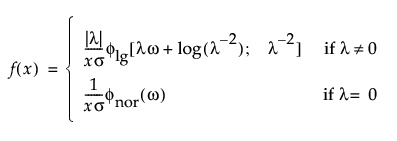where ω = [log(x) – μ]/σ. Note that the following is the pdf for the standardized log-gamma variable with shape parameter κ > 0: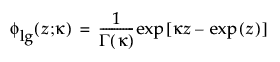Note that φnor(·) is the standard normal pdf.

Arguments

x

A quantile at which the pdf is evaluated. x must be greater than 0.

mu

The location parameter μ.

sigma

The scale parameter σ, which must be greater than 0.

lambda

A shape parameter λ.

GenGamma Distribution(x, mu, sigma, lambda)

Description

Returns the cumulative distribution function (cdf) of the extended generalized gamma distribution. The cdf is parameterized as follows: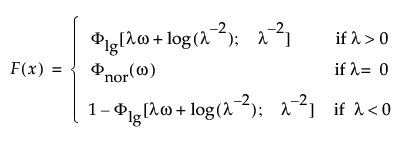where ω = [log(x) – μ]/σ. Note that the following is the cdf for the standardized log-gamma variable with shape parameter κ > 0: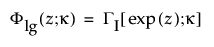where ΓI[·] denotes the incomplete gamma function. Note that Φnor(·) is the standard normal cdf.

Arguments

x

A quantile at which the cdf is evaluated. x must be greater than 0.

mu

The location parameter μ.

sigma

The scale parameter σ, which must be greater than 0.

lambda

A shape parameter λ.

GenGamma Quantile(p, mu, sigma, lambda)

Description

Returns the pth quantile from an extended generalized gamma distribution with parameters mu, sigma, and lambda. The quantile function does not have a closed form equation.

Arguments

p

The probability of the quantile desired. p must be between 0 and 1.

mu

The location parameter μ.

sigma

The scale parameter σ, which must be greater than 0.

lambda

A shape parameter λ.

GLog Density(x, mu, sigma, lambda)

Description

Returns the probability density function (pdf) evaluated at x of a generalized logarithmic distribution. The pdf is parameterized as follows: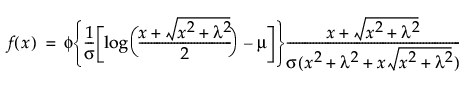where φ(·) is the standard normal pdf.

Arguments

x

A quantile at which the pdf is evaluated.

mu

The location parameter μ.

sigma

The scale parameter σ, which must be greater than 0.

lambda

A shape parameter λ, which must be greater than 0.

Notes

When the shape parameter is equal to zero, the distribution reduces to a Lognormal(μ, σ).

GLog Distribution(x, mu, sigma, lambda)

Description

Returns the probability that a generalized logarithmically distribution random variable is less than x. The cdf is parameterized as follows: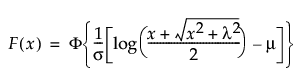where Φ(·) is the standard normal cdf.

Arguments

x

A quantile at which the cdf is evaluated.

mu

The location parameter μ.

sigma

The scale parameter σ, which must be greater than 0.

lambda

A shape parameter λ, which must be greater than 0.

GLog Quantile(p, mu, sigma, lambda)

Description

Returns the pth quantile from a generalized logarithmic distribution.

IGamma()

Johnson Sb Density(q, gamma, delta, theta, sigma)

Description

Returns the probability density function (pdf) evaluated at q of a Johnson Sb distribution. The pdf is parameterized as follows: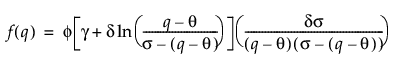where φ(·) is the standard normal pdf.

Arguments

q

A quantile at which the pdf is evaluated. q must be in the interval theta to theta + sigma.

gamma

Shape parameter γ.

delta

Shape parameter δ, which must be greater than 0.

theta

Location parameter θ.

sigma

Scale parameter σ, which must be greater than 0.

Johnson Sb Distribution(q, gamma, delta, theta, sigma)

Description

Returns the cumulative distribution function (cdf) evaluated at q of a Johnson Sb distribution. The pdf is parameterized as follows: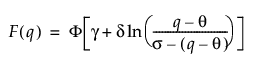where Φ(·) is the standard normal cdf.

Arguments

q

A quantile at which the cdf is evaluated. q must be in the interval theta to theta + sigma.

gamma

Shape parameter γ.

delta

Shape parameter δ, which must be greater than 0.

theta

Location parameter θ.

sigma

Scale parameter σ, which must be greater than 0.

Johnson Sb Quantile(p, gamma, delta, theta, sigma)

Description

Returns the pth quantile from a Johnson Sb distribution.

Arguments

p

The probability of the quantile desired. p must be between 0 and 1.

gamma

Shape parameter γ.

delta

Shape parameter δ, which must be greater than 0.

theta

Location parameter θ.

sigma

Scale parameter σ, which must be greater than 0.

Johnson Sl Density(x, gamma, delta, theta, sigma)

Description

Returns the probability density function (pdf) evaluated at x of a Johnson Sl distribution. The pdf is parameterized as follows: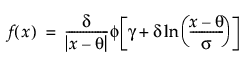where φ(·) is the standard normal pdf.

Arguments

x

A quantile at which the pdf is evaluated. x must be greater than theta if sigma is 1 and less than theta if sigma is -1.

gamma

Shape parameter γ.

delta

Shape parameter δ, which must be greater than 0.

theta

Location parameter θ.

sigma

Parameter σ that indicates if the distribution is skewed positively or negatively. sigma must be equal to either +1 (skewed positively) or -1 (skewed negatively).

Johnson Sl Distribution(q, gamma, delta, theta, sigma)

Description

Returns the cumulative distribution function (cdf) evaluated at q of a Johnson Sl distribution.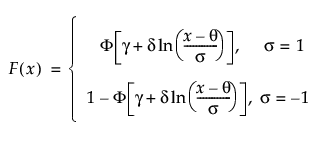where Φ(·) is the standard normal cdf.

Arguments

q

A quantile at which the cdf is evaluated. q must be greater than theta if sigma is 1 and less than theta if sigma is -1.

gamma

Shape parameter γ.

delta

Shape parameter δ, which must be greater than 0.

theta

Location parameter θ.

sigma

Parameter σ that defines if the distribution is skewed positively or negatively. Sigma must be equal to either +1 (skewed positively) or -1 (skewed negatively).

Johnson Sl Quantile(p, gamma, delta, theta, sigma)

Description

Returns the pth quantile from a Johnson Sl distribution.

Arguments

p

The probability of the quantile desired. p must be between 0 and 1.

gamma

Shape parameter γ.

delta

Shape parameter δ, which must be greater than 0.

theta

Location parameter θ.

sigma

Parameter σ that defines if the distribution is skewed positively or negatively. Sigma must be equal to either +1 (skewed positively) or -1 (skewed negatively).

Johnson Su Density(x, gamma, delta, theta, sigma)

Description

Returns the probability density function (pdf) evaluated at x of a Johnson Su distribution. The pdf is parameterized as follows: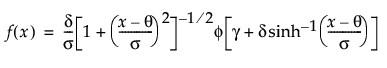where φ(·) is the standard normal pdf.

Arguments

x

A quantile at which the pdf is evaluated.

gamma

Shape parameter γ.

delta

Shape parameter δ, which must be greater than 0.

theta

Location parameter θ.

sigma

Scale parameter σ, which must be greater than 0.

Johnson Su Distribution(q, gamma, delta, theta, sigma)

Description

Returns the cumulative distribution function (cdf) evaluated at q of a Johnson Su distribution. The cdf is parameterized as follows: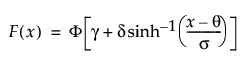where Φ(·) is the standard normal cdf.

Arguments

q

A quantile at which the cdf is evaluated.

gamma

Shape parameter γ.

delta

Shape parameter δ, which must be greater than 0.

theta

Location parameter θ.

sigma

Scale parameter σ, which must be greater than 0.

Johnson Su Quantile(p, gamma, delta, theta, sigma)

Description

Returns the pth quantile from a Johnson Su distribution.

Arguments

p

The probability of the quantile desired. p must be between 0 and 1.

gamma

Shape parameter γ.

delta

Shape parameter δ, which must be greater than 0.

theta

Location parameter θ.

sigma

Scale parameter σ, which must be greater than 0.

LEV Density(x, mu, sigma)

Description

Returns the probability density function (pdf) evaluated at x of the largest extreme value distribution with location mu and scale sigma. The pdf is parameterized as follows: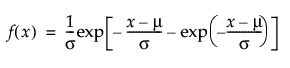Arguments

x

A quantile at which the pdf is evaluated.

mu

The location parameter μ.

sigma

The scale parameter σ, which must be greater than 0.

LEV Distribution(x, mu, sigma)

Description

Returns the cumulative distribution function (cdf) evaluated at x of the largest extreme value distribution with location mu and scale sigma. The cdf is parameterized as follows: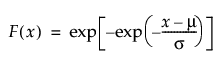Arguments

x

A quantile at which the cdf is evaluated. x must be greater than sigma.

mu

The location parameter μ.

sigma

The scale parameter σ, which must be greater than 0.

LEV Quantile(p, mu, sigma)

Description

Returns the pth quantile from a largest extreme value distribution with location mu and scale sigma. The quantile function is parameterized as follows: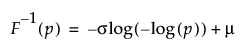Arguments

p

The probability of the quantile desired. p must be between 0 and 1.

mu

The location parameter μ.

sigma

The scale parameter σ, which must be greater than 0.

LogGenGamma Density(x, mu, sigma, lambda)

Description

Returns the probability density function (pdf) evaluated at x of a log generalized gamma probability distribution with parameters mu, sigma, and lambda. The pdf is parameterized as follows: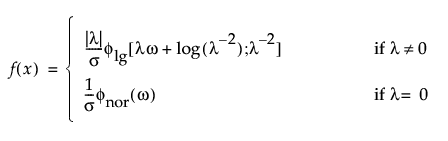where ω = [xμ]/σ. Note that the following is the pdf for the log-gamma variable with shape parameter κ > 0:Note that φnor(·) is the standard normal pdf.

Arguments

x

A quantile at which the pdf is evaluated.

mu

The location parameter μ.

sigma

The scale parameter σ, which must be greater than 0.

lambda

A shape parameter λ.

LogGenGamma Distribution(x, mu, sigma, lambda)

Description

Returns the cumulative distribution function (cdf) evaluated at x of the log generalized gamma distributed random variable (with parameters mu, sigma, and lambda) The cdf is parameterized as follows: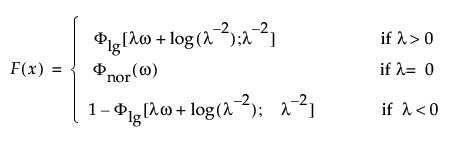where ω = [xμ]/σ. Note that the following is the cdf for the log-gamma variable with shape parameter κ > 0:where ΓI[·] denotes the incomplete gamma function. Note that Φnor(·) is the standard normal cdf.

Arguments

x

A quantile at which the cdf is evaluated.

mu

The location parameter μ.

sigma

The scale parameter σ, which must be greater than 0.

lambda

A shape parameter λ.

LogGenGamma Quantile(p, mu, sigma, lambda)

Description

Returns the pth quantile from a log generalized gamma distribution.

Arguments

p

The probability of the quantile desired. p must be between 0 and 1.

mu

The location parameter μ.

sigma

The scale parameter σ, which must be greater than 0.

lambda

A shape parameter λ.

Logistic Density(x, mu, sigma)

Description

Returns the probability density function (pdf) evaluated at x of a logistic distribution with location mu and scale sigma. The pdf is parameterized as follows: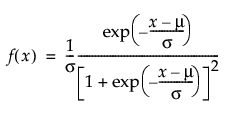Arguments

x

A quantile at which the pdf is evaluated.

mu

The location parameter μ.

sigma

The scale parameter σ, which must be greater than 0.

Logistic Distribution(x, mu, sigma)

Description

Returns the cumulative distribution function (cdf) evaluated at x of the logistic distribution with location mu and scale sigma. The cdf is parameterized as follows: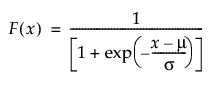Arguments

x

A quantile at which the cdf is evaluated. x must be greater than σ.

mu

The location parameter μ.

sigma

The scale parameter σ, which must be greater than 0.

Logistic Quantile(p, mu, sigma)

Description

Returns the pth quantile from a logistic distribution with location mu and scale sigma. The quantile function is parameterized as follows: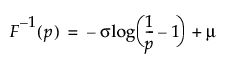Arguments

p

The probability of the quantile desired. p must be between 0 and 1.

mu

The location parameter μ.

sigma

The scale parameter σ, which must be greater than 0.

Loglogistic Density(x, mu, sigma)

Description

Returns the probability density function (pdf) evaluated at x of a loglogistic distribution with location mu and scale sigma. The pdf is parameterized as follows: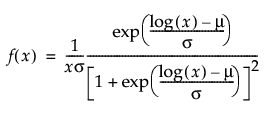Arguments

x

A quantile at which the pdf is evaluated.

mu

The location parameter μ.

sigma

The scale parameter σ, which must be greater than 0.

Loglogistic Distribution(x, mu, sigma)

Description

Returns the cumulative distribution function (cdf) evaluated at x of the loglogistic distribution with location mu and scale sigma. The cdf is parameterized as follows: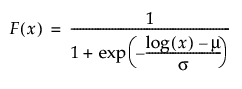Arguments

x

A quantile at which the cdf is evaluated.

mu

The location parameter μ.

sigma

The scale parameter σ, which must be greater than 0.

Loglogistic Quantile(p, mu, sigma)

Description

Returns the pth quantile from a loglogistic distribution with location mu and scale sigma. The quantile function is parameterized as follows: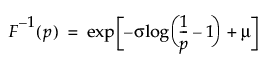Arguments

p

The probability of the quantile desired. p must be between 0 and 1.

mu

The location parameter μ.

sigma

The scale parameter σ, which must be greater than 0.

Lognormal Density(x, mu, sigma)

Description

Returns the probability density function (pdf) evaluated at x of a lognormal distribution with location mu and scale sigma. The pdf is parameterized as follows: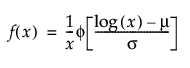where φ(·) is the standard normal pdf.

Arguments

x

A quantile at which the pdf is evaluated. x must be greater than or equal to 0.

mu

The location parameter μ.

sigma

The scale parameter σ, which must be greater than 0.

Lognormal Distribution(x, mu, sigma)

Description

Returns the cumulative distribution function (cdf) evaluated at x of a lognormal distribution with location mu and scale sigma. The cdf is parameterized as follows: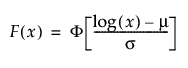where Φ(·) is the standard normal cdf.

Arguments

x

A quantile at which the pdf is evaluated. x must be greater than or equal to 0.

mu

The location parameter μ.

sigma

The scale parameter σ, which must be greater than 0.

Lognormal Quantile(x, mu, sigma)

Description

Returns the pth quantile of a lognormal distribution with location mu and scale sigma.

Normal Biv Distribution(x, y, r, <mu1>, <s1>, <mu2>, <s2>)

Description

Computes the probability that an observation (X, Y) is less than or equal to (x, y) with correlation coefficient r where X is individually normally distributed with mean mu1 and standard deviation s1 and Y is individually normally distributed with mean mu2 and standard deviation s2. If mu1, s1, mu2, and s2 are not given, the function assumes the standard normal bivariate distribution with mu1=0, s1=1, mu2=0, and s2=1.

Normal Density(x, <mean=0>, <stddev=1>)

Description

Returns the probability density function (pdf) evaluated at x for the normal distribution with mean and stddev. The pdf is parameterized as follows: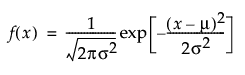Arguments

x

A quantile at which the pdf is evaluated.

mu

Optional location parameter μ. The default is 0.

sigma

Optional scale parameter σ, which must be greater than 0. The default is 1.

Notes

The normal distribution is bell shaped and symmetrical.

Normal Distribution(x, <mean=0>, <stddev=1>)

Description

Returns the cumulative distribution function (cdf) evaluated at x for the normal distribution with mean and stddev. The cdf is parameterized as follows: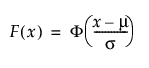Note that Φ(·) is the standard normal cdf, defined as follows: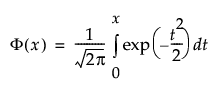Arguments

x

A quantile at which the pdf is evaluated.

mu

Optional location parameter μ. The default is 0.

sigma

Optional scale parameter σ, which must be greater than 0. The default is 1.

Normal Log CDistribution(x, <mean=0>, <std dev=1>)

Description

Returns 1 - log (value) of the distribution function at quantile x for the normal distribution.

Normal Log Density(x, <mean=0>, <stddev=1>)

Description

Returns the log of the value of the density function at quantile x for the normal distribution with mean and stddev. The default mean is 0. The default stddev is 1.

Normal Log Distribution(x, <mean=0>, <std dev=1>)

Description

Returns the log of the value of the distribution function at quantile x for the normal distribution.

Normal Mixture Density(q, mean, stdev, probability)

Description

Returns the density at q of a normal mixture distribution with group means mean, group standard deviations stdev, and group probabilities probability. The mean, stdev, and probability arguments are all vectors of the same size.

Normal Mixture Distribution(q, mean, stdev, probability)

Description

Returns the probability that a normal mixture distributed variable with group means mean, group standard deviations stdev, and group probabilities probability is less than q. The mean, stdev, and probability arguments are all vectors of the same size.

Normal Mixture Quantile(p, mean, stdev, probability)

Description

Returns the pth quantile, the values for which the probability is p that a random value would be lower. The mean, stdev, and probability arguments are all vectors of the same size.

Normal Quantile(p, <mean=0>, <stddev=1>)

Probit(p, <mean=0>, <stddev=1>)

Description

Returns the pth quantile from the normal distribution with mean and stddev. The default mean is 0. the default stddev is 1.

Probit()

SEV Density(x, mu, sigma)

Description

Returns the probability density function (pdf) evaluated at x of the smallest extreme distribution with location mu and scale sigma. The pdf is parameterized as follows: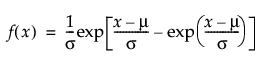Arguments

x

A quantile at which the pdf is evaluated.

mu

The location parameter μ.

sigma

The scale parameter σ, which must be greater than 0.

SEV Distribution(x, mu, sigma)

Description

Returns the cumulative distribution function (cdf) evaluated at x of the smallest extreme distribution with location mu and scale sigma. The cdf is parameterized as follows: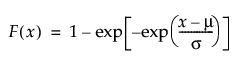Arguments

x

A quantile at which the cdf is evaluated. x must be greater than σ.

mu

The location parameter μ.

sigma

The scale parameter σ, which must be greater than 0.

SEV Quantile(p, mu, sigma)

Description

Returns the pth quantile of the smallest extreme distribution with location mu and scale sigma. The quantile function is parameterized as follows: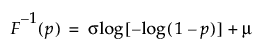Arguments

p

The probability of the quantile desired. p must be between 0 and 1.

mu

The location parameter μ.

sigma

The scale parameter σ, which must be greater than 0.

SHASH Density(x, gamma, delta, theta, sigma)

Description

Returns the probability density function (pdf) evaluated at x of a sinh-arcsinh (SHASH) distribution. The pdf is parameterized as follows: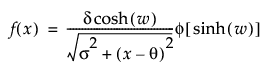where

φ(·) is the standard normal pdf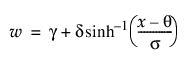Arguments

x

A quantile at which the pdf is evaluated.

gamma

The shape parameter γ.

delta

The shape parameter δ, which must be greater than 0.

theta

The location parameter θ.

sigma

The scale parameter σ, which must be greater than 0.

SHASH Distribution(x, gamma, delta, theta, sigma)

Description

Returns the cumulative distribution function (cdf) evaluated at x of the sinh-arcsinh (SHASH) distribution. The cdf is parameterized as follows: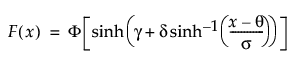where Φ(·) is the standard normal cdf.

Arguments

x

A quantile at which the cdf is evaluated.

gamma

The shape parameter γ.

delta

The shape parameter δ, which must be greater than 0.

theta

The location parameter θ.

sigma

The scale parameter σ, which must be greater than 0.

SHASH Quantile(p, gamma, delta, theta, sigma)

Description

Returns the pth quantile from a sinh-arcsinh (SHASH) distribution (with parameters gamma, delta, theta, and sigma).

Arguments

p

The probability of the quantile desired. p must be between 0 and 1.

gamma

The shape parameter γ.

delta

The shape parameter δ, which must be greater than 0.

theta

The location parameter θ.

sigma

The scale parameter σ, which must be greater than 0.

Students t Density()

Students t Distribution()

Students t Quantile()

t Density(x, df, <nc=0>)

Students t Density(x, df, <nc=0>)

Description

Returns the probability density function (pdf) evaluated at x of the Student’s t distribution with degrees of freedom df. The pdf is parameterized as follows: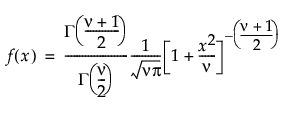Arguments

x

A quantile at which the pdf is evaluated.

df

The degrees of freedom ν, which must be greater than or equal to 1.

nc

The optional noncentrality parameter λ, which must be nonnegative. The default is 0.

t Distribution(q, df, <nc=0>)

Students t Distribution(q, df, <nc=0>)

Description

Returns the probability that a Student’s t distributed random variable is less than q. nc defaults to 0.

t Log CDistribution(x, df, <nc=0>)

Description

Returns 1 - log (value) of the normal distribution function at quantile x for the t distribution.

t Log Density(x, df, <nc=0>)

Description

Returns the log of the value of the density function at quantile x for the t distribution.

t Log Distribution(x, df, <nc=0>)

Description

Returns the log of the value of the distribution function at quantile x for the t distribution.

t Noncentrality(x, df, prob)

Description

Returns the t distribution noncentrality parameter nc that satisfies the following:

prob = T Distribution(x, df, nc)

t Quantile(p, df, <nc=0>)

Students t Quantile(p, df, <nc=0>)

Description

Returns the pth quantile from the Student’s t distribution with degrees of freedom df. nc defaults to 0.

Tukey HSD P Value(q, n, dfe)

Description

Returns the p-value from Tukey’s HSD multiple comparisons test.

Arguments

q

The test statistic. The test statistic that is specified is Tukey’s adjusted critical value, which is the quantile of Tukey’s studentized range distribution divided by the square root of 2.

n

The number of groups in the study.

dfe

The error degrees of freedom, based on the total study sample.

Tukey HSD Quantile(1-alpha, n, dfe)

Description

Returns the quantile used in Tukey’s HSD multiple comparisons test. The quantile that is returned is Tukey’s adjusted critical value, which is the quantile of Tukey’s studentized range distribution divided by the square root of 2.

Arguments

1-alpha

The confidence level.

n

The number of groups in the study.

dfe

The error degrees of freedom, based on the total study sample.

Weibull Density(x, shape, <scale=1>, <threshold=0>)

Description

Returns the probability density function (pdf) evaluated at x of the Weibull distribution. The pdf is parameterized as follows: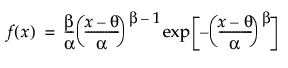Arguments

x

A quantile the pdf is evaluated at. x must be greater than threshold.

shape

Shape parameter β, which must be greater than 0.

scale

Optional scale parameter α, which must be greater than 0. The default is 1.

threshold

Optional threshold parameter θ. The default is 0.

Weibull Distribution(x, shape, <scale=1>, <threshold=0>)

Description

Returns the cumulative distribution function (cdf) at x of the Weibull distribution. The cdf is parameterized as follows: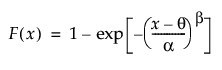Arguments

x

A quantile at which the pdf is evaluated. x must be greater than threshold.

shape

Shape parameter β, which must be greater than 0.

scale

Optional scale parameter α, which must be greater than 0. The default is 1.

threshold

Optional threshold parameter θ. The default is 0.

Weibull Quantile(p, shape, <scale=1>, <threshold=0>)

Description

Returns the pth quantile from the Weibull distribution with the parameters given. The quantile function is calculated as follows: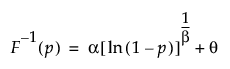Arguments

p

The probability of the quantile desired. p must be between 0 and 1.

shape

Shape parameter β, which must be greater than 0.

scale

Optional scale parameter α, which must be greater than 0. The default is 1.

threshold

Optional threshold parameter θ. The default is 0.